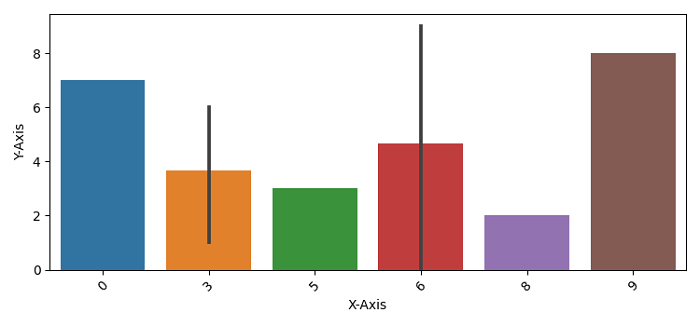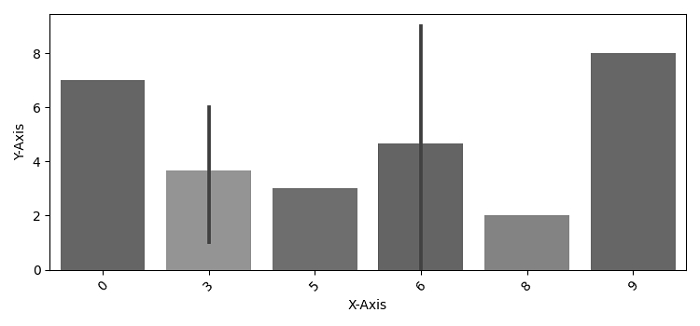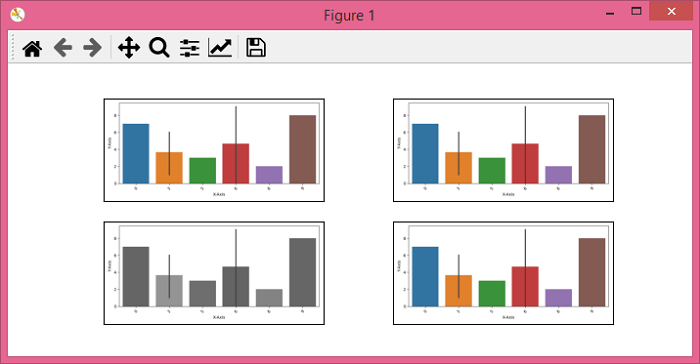# PyTorch – torchvision.transforms – RandomGrayscale()

PyTorchServer Side ProgrammingProgramming

To randomly convert an image to grayscale with a probability, we apply RandomGrayscale() transformation. It's one of the transforms provided by the torchvision.transforms module. This module contains many important transformations that can be used to perform different manipulations on the image data.

RandomGrayscale() accepts both PIL and tensor images or a batch of tensor images. A tensor image is a PyTorch Tensor with shape [3, H, W], where H is the image height and W is the image width. A batch of tensor images is also a torch tensor with [B, 3, H, W]. B is the number of images in the batch.

## Syntax

torchvision.transforms.RandomGrayscale(p)(img)
• If p = 1, it returns a grayscale image.

• If p = 0, it returns a original image.

• If p is in the range (0,1), then the probability to return a grayscale image is p. It returns a random grayscale image with a given probability p.

## Steps

We could use the following steps to randomly convert an image to grayscale with a given probability −

• Import the required libraries. In all the following examples, the required Python libraries are torch, Pillow, and torchvision. Make sure you have already installed them.

import torch
import torchvision
import torchvision.transforms as T
from PIL import Image
• Read the input image. The input image is a PIL image or a torch tensor.

img = Image.open('bargraph.png')
• Define a transform to randomly convert the original input image to grayscale with a given probability p.

transform = T.RandomGrayscale(p = 0.25)
• Apply the above-defined transform on the input image to convert it to grayscale.

img = transform(img)
• Show the grayscale image.

img.show()

## Input Image

This image is used as the input in all the following examples.## Example 1

In this Python3 program, we use p = 1. It definitely converts the image to grayscale.

# import required libraries
import torch
import torchvision.transforms as T
from PIL import Image

# read the input image
img = Image.open('bargraph.png')

# define the transform to randomly convert the input image
# to grayscale with a probability
transform = T.RandomGrayscale(p=1)

# apply the above transform on input image
img = transform(img)
img.show()

## Output

It will produce the following output −## Example 2

In this program, we use p = 0.25% This means, there is a 25% chance of an image to be converted to grayscale.

import torch
import torchvision.transforms as T
from PIL import Image
import matplotlib.pyplot as plt
img = Image.open('bargraph.png')
transform = T.RandomGrayscale(p=0.25)

imgs = [transform(img) for _ in range(4)]

fig = plt.figure(figsize=(7,3))
rows, cols = 2,2
for j in range(0, len(imgs)):
plt.imshow(imgs[j])
plt.xticks([])
plt.yticks([])
plt.show()

## Output

It will produce the following output −Notice that out of 4 output images, one image is in grayscale. This is because we have set p = 0.25.